Courses

# Resultants of a Force and Couple System Mechanical Engineering Notes | EduRev

## Mechanical Engineering : Resultants of a Force and Couple System Mechanical Engineering Notes | EduRev

The document Resultants of a Force and Couple System Mechanical Engineering Notes | EduRev is a part of the Mechanical Engineering Course Engineering Mechanics - Notes, Videos, MCQs & PPTs.
All you need of Mechanical Engineering at this link: Mechanical Engineering

The basic idea: Two force systems are equivalent if they result in the same resultant force and the same resultant moment.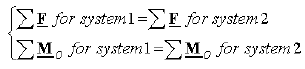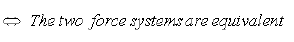Moving a force along its line of action: Moving a force along its line of action results in a new force system which is equivalent to the original force system.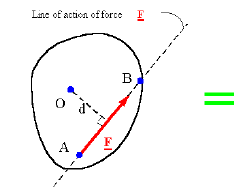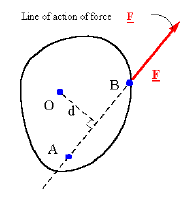Moving a force off its line of action: If a force is moved off its line of action, a couple must be added to the force system so that the new system generates the same moment as the old system.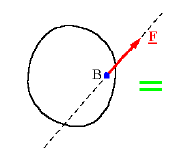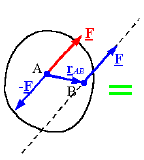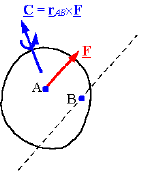The resultant of a force and couple system: For any point O, every force and couple system can be made equivalent to a single force passing through O and a single couple. The single force passing through O is equal to the resultant force of the original system, and the couple is equal to the resultant moment of the original system around point O.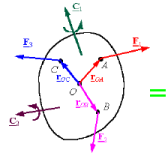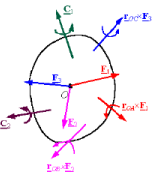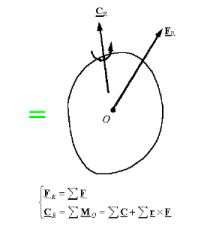When can one reduce a force and couple system to a single force?: For a force and couple system if the resultant force and the resultant couple are perpendicular, then one can find an equivalent system with a single force and no couple. To obtain this system, move the resultant force a distance d along the line perpendicular to the plane of the resultant force and resultant couple until the resultant force creates a moment equivalent to the resultant couple.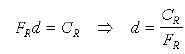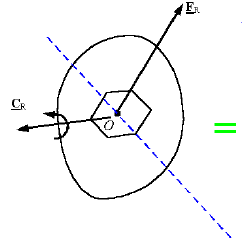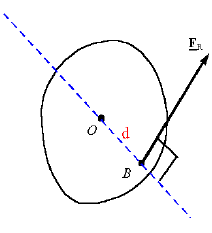Note: All 2-D force systems can be reduced to a single force. To find the line of action of the force, the moment of the original system must be forced to be the same as the system with the single force.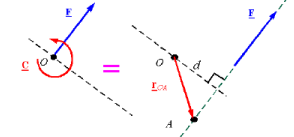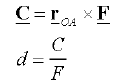Offer running on EduRev: Apply code STAYHOME200 to get INR 200 off on our premium plan EduRev Infinity!

## Engineering Mechanics - Notes, Videos, MCQs & PPTs

30 videos|72 docs|65 tests

,

,

,

,

,

,

,

,

,

,

,

,

,

,

,

,

,

,

,

,

,

;